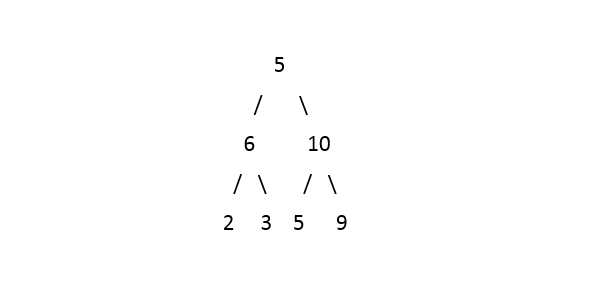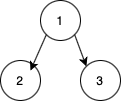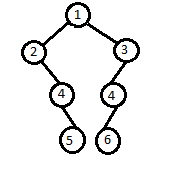Problem title
Difficulty
Avg time to solve

Postorder Successor
Moderate
15 mins
Easy
15 mins
Reverse DLL nodes in groups
Moderate
10 mins
Find Node
Moderate
25 mins
Normal BST To Balanced BST
Moderate
15 mins
Find K-th Row
Easy
15 mins
Count Subtrees
Easy
15 mins
Path Reversals
Moderate
25 mins
Zigzag Binary Tree Traversal
Easy
10 mins
Boredom
Moderate
25 mins6

# Deepest Right Leaf

Difficulty: MEDIUM
Avg. time to solve
20 min
Success Rate
75%

Problem Statement

#### You have been given a Binary Tree of 'N' nodes. Your task is to find and return the deepest right leaf node. In case of multiple answers, return the rightmost node.

##### For example:
``````For the given binary tree:
````````````Output: 9

Explanation: The deepest right nodes are 3 and 9 but 9 is the rightmost, thus 9 is the answer.
``````
##### Input Format:
``````The only line of the input contains elements in the level order form. The line consists of values of nodes separated by a single space. In case a node is null, we take -1 in its place.
``````
##### Example:
``````The input for the tree depicted in the below image would be :
1 2 3 4 -1 5 6 -1 7 -1 -1 -1 -1 -1 -1
``````#### Explanation :

``````Level 1 :
The root node of the tree is 1

Level 2 :
Left child of 1 = 2
Right child of 1 = 3

Level 3 :
Left child of 2 = 4
Right child of 2 = null (-1)
Left child of 3 = 5
Right child of 3 = 6

Level 4 :
Left child of 4 = null (-1)
Right child of 4 = 7
Left child of 5 = null (-1)
Right child of 5 = null (-1)
Left child of 6 = null (-1)
Right child of 6 = null (-1)

Level 5 :
Left child of 7 = null (-1)
Right child of 7 = null (-1)

1
2 3
4 -1 5 6
-1 7 -1 -1 -1 -1
-1 -1

The first not-null node (of the previous level) is treated as the parent of the first two nodes of the current level. The second not-null node (of the previous level) is treated as the parent node for the next two nodes of the current level and so on.
``````
##### Note :
``````1. The above format was just to provide clarity on how the input is formed for a given tree.

2. The input ends when all nodes at the last level are null (-1).
``````
##### Output Format:
``````The only line of output will contain an integer representing the deepest right leaf node of the given binary tree.
``````
##### Note:
``````You don't need to print the output, it has already been taken care of. Just implement the given function.
``````
##### Constraints:
``````0 <= N <= 10^5
0 <= data <= 10^5

Where 'N' denotes the number of nodes in the Binary Tree and 'data' represents the node value of the nodes in the Binary Tree.

Time limit: 1 sec
``````
##### Sample Input 1:
``````1 2 3 -1 -1 -1 -1
``````
##### Sample Output 1:
``````3
``````
##### Explanation to Sample Output 1:
``````The input binary tree will be represented as:
````````````From the above representation, the leaves node are 2 and 3. But 3 is the right child of its parent but 2 is the left child of its parent. Thus, 3 should be the answer.
``````
##### Sample Input 2:
``````1 2 3 -1 4 4 -1 -1 5 6 -1 -1 -1 -1 -1
``````
##### Sample Output 2:
``````5
``````
##### Explanation to Sample Output 2:
``````The input binary tree will be represented as:
````````````From the above representation, the leaves node are 5 and 6. But 5 is the right child of its parent but 6 is the left child of its parent. Thus, 5 should be the answer.
``````Console